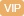| APP | 校园号 | 客服 客服热线：400-863-9889

400-863-98893 5
×20
=
10×
 1 5
=
 7 18
×9
=
15×
 3 5
=
 4 7
+
 5 7
=
 5 6
=
 3 5
×5
=
 5 9
×15
=
【考点】分数乘法【专题】计算题．
【分析】小数化分数：原来有几位小数，就1的写个零作分母，把原来小掉小数点作子，能约分的要约分，依此解．
【解答】解：0.=
 3 10
1.=1
 5 10
=1
 5÷5 0÷5
=1
 1 2
；             .2=3
 25 100
=3
 2525 10÷25
=3
 1 4
【点评】考查了数与分的互化，键是熟练掌握计算法则正进算．

0/0